# What is the electric field strength inside the capacitor?

What is the electric field strength inside the capacitor?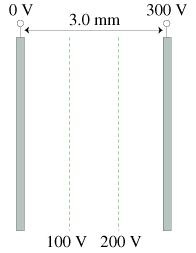What is the potential energy of a proton at the midpoint of the capacitor?

Concepts and reason
The concept used to solve this problem is electric field strength and potential energy.

Initially, the electric field strength can be calculated by using the relation between electric field, potential and the distance between the capacitor. Later, the potential energy of a proton at the midpoint can be calculated by using the relation between the charge of proton and the potential.

Fundamentals
The expression for the electric field strength is,Here, is the electric field strength,V is the potential, and d is the distance between the capacitor.
The expression of the potential energy at the midpoint is,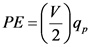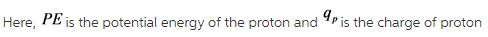The expression for the electric field strength is,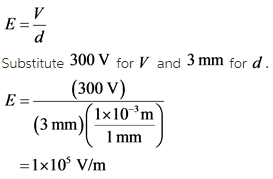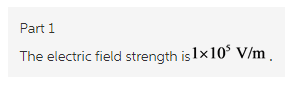Explanation:
The electric field strength is calculated by substituting the value of the potential and the separation distance between the capacitor. The unit of the separation distance is converted by using the unit conversion method.

(2)
The expression for the potential energy is,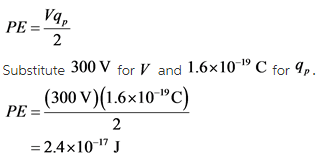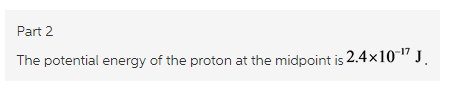Explanation:
The potential energy is calculated by substituting the value of the potential and the charge of proton.